{{ message }}

tannerlinsley / react-ranger Public

⚛️ Hooks for building range and multi-range sliders in React

Switch branches/tags
Nothing to show

Files

Failed to load latest commit information.
Type
Name
Commit timeHooks for building range and multi-range sliders in ReactEnjoy this library? Try them all! React Table, React Query, React Form, React Charts

Examples

This library is being built and maintained by me, @tannerlinsley and I am always in need of more support to keep projects like this afloat. If you would like to get premium support, add your logo or name on this README, or simply just contribute to my open source Sponsorship goal, visit my Github Sponsors page!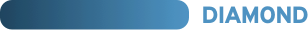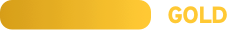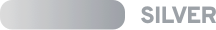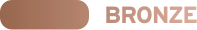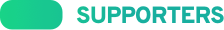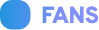Installation

\$ npm i --save react-ranger
# or

Sample Usage

The following is a very basic example of a single range input that looks similar to Chrome's default appearance.

import ReactRanger from 'react-ranger'

function App() {
const [values, setValues] = React.useState()

const { getTrackProps, handles } = useRanger({
values,
onChange: setValues,
min: 0,
max: 100,
stepSize: 5,
})

return (
<>
<div
{...getTrackProps({
style: {
height: '4px',
background: '#ddd',
boxShadow: 'inset 0 1px 2px rgba(0,0,0,.6)',
},
})}
>
{handles.map(({ getHandleProps }) => (
<div
{...getHandleProps({
style: {
width: '12px',
height: '12px',
background: 'linear-gradient(to bottom, #eee 45%, #ddd 55%)',
border: 'solid 1px #888',
},
})}
/>
))}
</div>
</>
)
}

Options

• value: Array<number> - The current value (or values) for the range
• Required
• min: number - The minimum limit for the range
• Required
• max: number - The maximum limit for the range
• Required
• stepSize: number - The distance between selectable steps
• Required
• steps: arrayOf(number) - An array of custom steps to use. This will override stepSize,
• tickSize: number
• ticks: arrayOf(number): Default: 10 - An array of custom ticks to use. This will override tickSize,
• onChange: Function(newValue) - A function that is called when the handle is released
• onDrag: Function(newValue) - A function that is called when a handled is dragged
• interpolator: { getPercentageForValue: Function(value) => decimal, getValueForClientX: Function(x) => value}
• The Interpolator to use
• Defualts to the bundled linear-scale interpolator See the Interpolation section for more info

Returns

useRanger returns an object with the following properties:

• getTrackProps(userProps): func - A function that takes optional props and returns the combined necessary props for the track component.
• ticks: array - Ticks to be rendered. Each tick has the following props:
• value: number - The tick number to be displayed
• getTickProps(userProps): func - A function that take optional props and returns the combined necessary props for the tick component.
• segments: array - Segments to be rendered. Each segment has the following props:
• value: number - The segments ending value
• getSegmentProps(userProps): func - A function that take optional props and returns the combined necessary props for the segment component.
• handles: array - Handles to be rendered. Each handle has the following props:
• value: number - The current value for the handle
• active: boolean - Denotes if the handle is currently being dragged.
• getHandleProps(userProps): func - A function that take optional props and returns the combined necessary props for the handle component.
• activeHandleIndex: oneOfType([null, number]) - The zero-based index of the handle that is currently being dragged, or null if no handle is being dragged.

Interpolation

By default, react-ranger uses linear interpolation between data points, but allows you to easily customize it to use your own interpolation functions by passing an object that implements the following interface:

const interpolator = {
// Takes the value & range and returns a percentage [0, 100] where the value sits from left to right
getPercentageForValue: (val: number, min: number, max: number): number

// Takes the clientX (offset from the left edge of the ranger) along with the dimensions
// and range settings and transforms a pixel coordinate back into a value
getValueForClientX: (clientX: number, trackDims: object, min: number, max: number): number
}

Here is an exmaple of building and using a logarithmic interpolator!

import { useRanger } from 'react-ranger'

getPercentageForValue: (val, min, max) => {
const minSign = Math.sign(min)
const maxSign = Math.sign(max)

if (minSign !== maxSign) {
throw new Error(
'Error: logarithmic interpolation does not support ranges that cross 0.'
)
}

let percent =
(100 / (Math.log10(Math.abs(max)) - Math.log10(Math.abs(min)))) *
(Math.log10(Math.abs(val)) - Math.log10(Math.abs(min)))

if (minSign < 0) {
// negative range, means we need to invert our percent because of the Math.abs above
return 100 - percent
}

return percent
},
getValueForClientX: (clientX, trackDims, min, max) => {
const { left, width } = trackDims
let value = clientX - left
value *= Math.log10(max) - Math.log10(min)
value /= width
value = Math.pow(10, Math.log10(min) + value)
return value
},
}

useRanger({
})

Contributors ✨

Thanks goes to these wonderful people (emoji key):

This project follows the all-contributors specification. Contributions of any kind welcome!

⚛️ Hooks for building range and multi-range sliders in React

v2.1.0 Latest
Sep 11, 2020